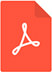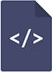# Effect of correlated measurement errors on estimation of population mean with modified ratio estimator

Biometrics & Biostatistics International Journal
Okafor Ikechukwu Boniface, Onyeka Aloysius Chijioke, Ogbonna Chukwudi Justin, Izunobi Chinyeaka HostensiaPDF Full Text

# Abstract

This paper proposes a class of modified ratio estimators of population mean using correlation coefficient between study and auxiliary variables in the presence of correlated measurement errors under simple random strategy. Usual unbiased estimator of sample mean per unit, ratio and product-type estimators belong to the suggested modified class of estimators. Considering large sample approximation, properties of the proposed estimator are obtained. Theoretical and empirical analysis revealed that the proposed class of estimators are more efficient than some existing estimators.

# Keywords

Correlated measurement errors, ratio estimator, bias, mean squared error, correlation coefficient